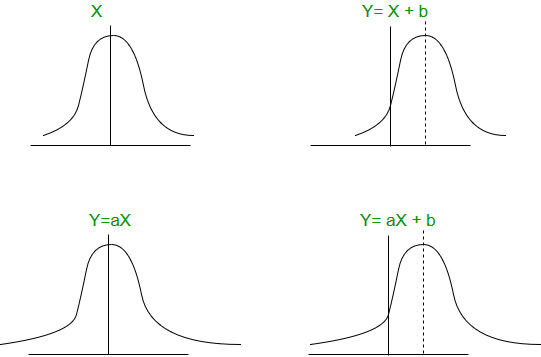GFG App
Open AppBrowser
Continue

# GATE | GATE CS 2011 | Question 33

Consider a finite sequence of random values X = { x1, x2,…, xn}. Let μx be the mean and σx be the standard deviation of X. Let another finite sequence Y of equal length be derived from this as yi = a*xi + b, where a and b are positive constants. Let μy be the mean and σy be the standard deviation of this sequence. Which one of the following statements is INCORRECT?
(A) Index position of mode of X in X is the same as the index position of mode of Y in Y.
(B) Index position of median of X in X is the same as the index position of median of Y in Y.
(C) μy = aμx+b
(D) σy = aσx+b

Explanation: Adding a constant like b shift the distribution while multiplying to a constant like a stretch the distribution along medianMode is the most frequent data of the distribution, so the index position of the mode will not change. From the above graph it is clear that index position of the median will also not change. Now for the meanAnd for the standard deviationMy Personal Notes arrow_drop_up